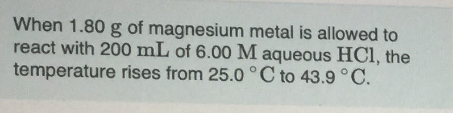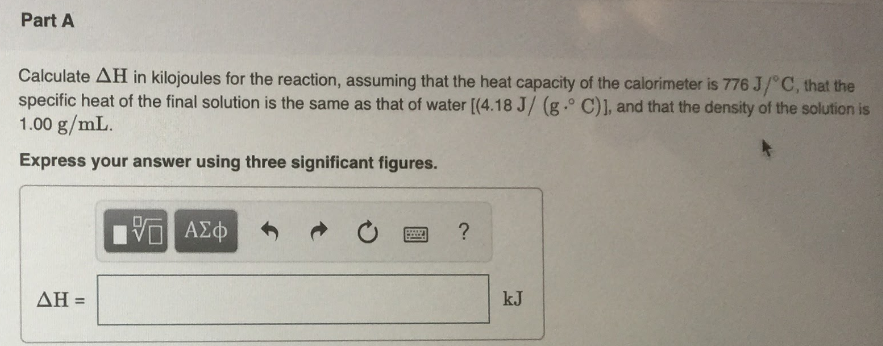# When 1.80 g of magnesium metal is allowed to react with 200 mL of 6.00 M aqueous HCl, the temperature rises from 25.0°C to 43.9°C. Calculate ΔH in kilojoules for the reaction, assuming that the heat capacity of the calorimeter is 776 J/°C, that the specific heat of the final solution is the same as that of water [4.18 J/ (g . °C)], and that the density of the solution is 1.00 g/mL. Express your answer using three significant figures.